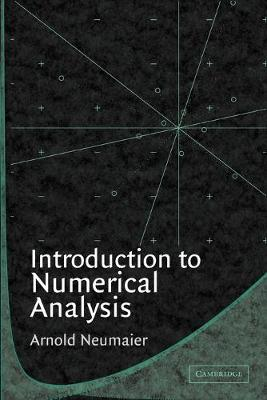•# Introduction to Numerical Analysis (Paperback)

(author)
£58.00
Paperback 366 Pages / Published: 01/10/2001
• We can order this

Usually dispatched within 3 weeks

Numerical analysis is an increasingly important link between pure mathematics and its application in science and technology. This textbook provides an introduction to the justification and development of constructive methods that provide sufficiently accurate approximations to the solution of numerical problems, and the analysis of the influence that errors in data, finite-precision calculations, and approximation formulas have on results, problem formulation and the choice of method. It also serves as an introduction to scientific programming in MATLAB, including many simple and difficult, theoretical and computational exercises. A unique feature of this book is the consequent development of interval analysis as a tool for rigorous computation and computer assisted proofs, along with the traditional material.

Publisher: Cambridge University Press
ISBN: 9780521336109
Number of pages: 366
Weight: 540 g
Dimensions: 229 x 152 x 21 mm

MEDIA REVIEWS
'... very valuable in preparing a course on Numerical Analysis and is indeed very readable.' Thomas Sonar, Zentralblatt MATH
'This is very strongly recommended reading for all undergraduate students whose courses require a serious understanding and implementation of numerical analysis.' The Mathematical Gazette